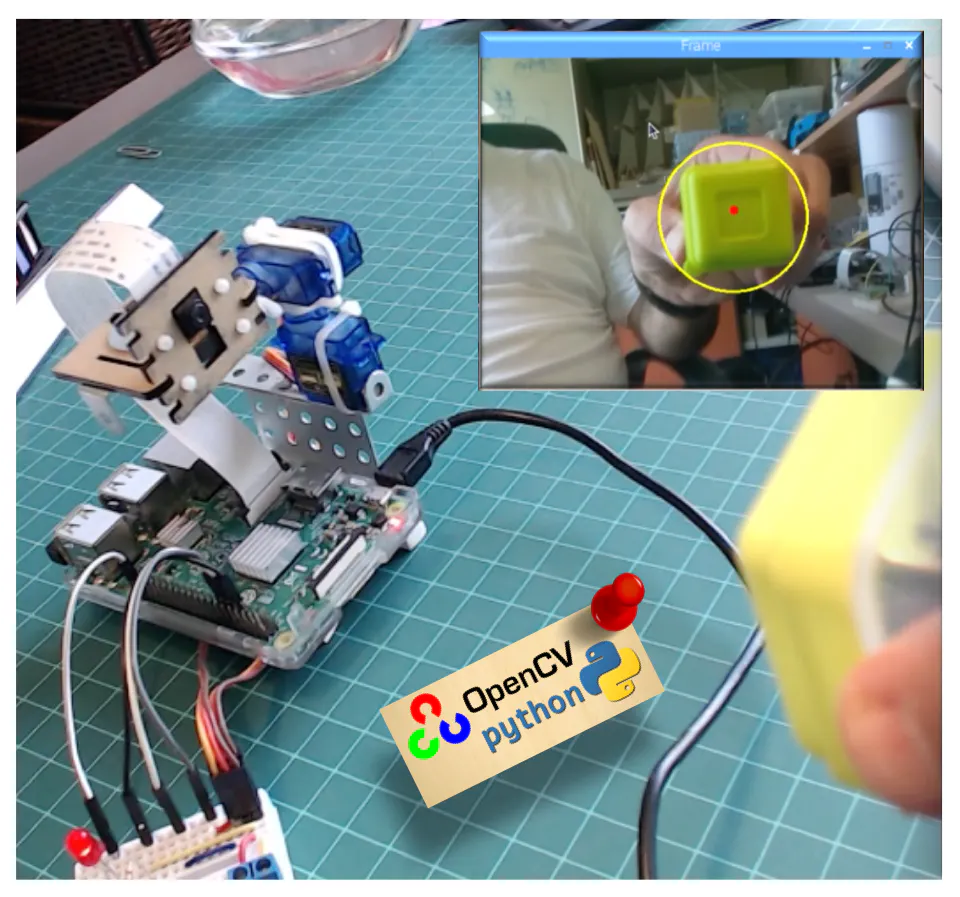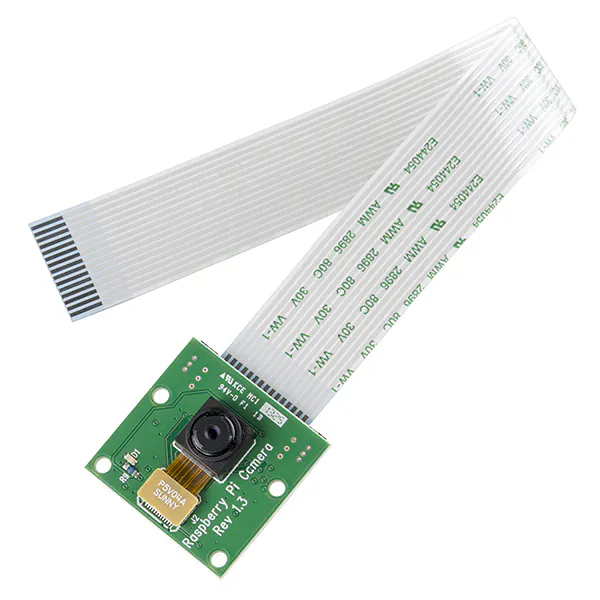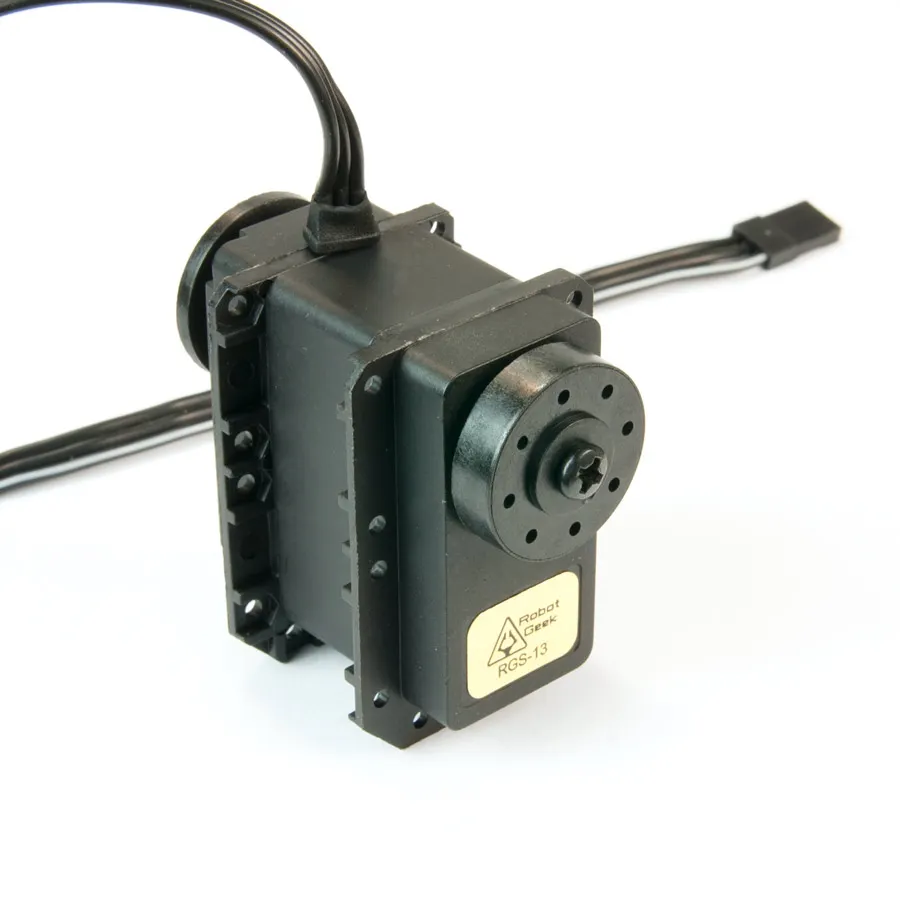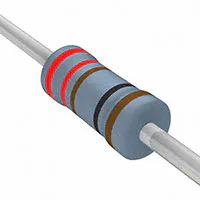# Automatic Vision Object Tracking

A pan/tilt servo device helping a camera to automatically track color objects using vision.

IntermediateFull instructions provided12 hours35,110## Things used in this project

### Hardware componentsRaspberry Pi 3 Model B
×1Raspberry Pi Camera Module
×1RobotGeek 180 Degree Robot Servo
×2LED (generic)
×1Resistor 221 ohm
×1

### Software apps and online services

 Raspberry Pi Raspbian OS (Stretch)OpenCV

## Code

### Code snippet #7

Plain text
```import numpy as np
import cv2

cap = cv2.VideoCapture(0)

while(True):
frame = cv2.flip(frame, -1) # Flip camera vertically
gray = cv2.cvtColor(frame, cv2.COLOR_BGR2GRAY)

cv2.imshow('frame', frame)
cv2.imshow('gray', gray)
if cv2.waitKey(1) & 0xFF == ord('q'):
break

cap.release()
cv2.destroyAllWindows()
```

### Code snippet #9

Plain text
```import sys
import numpy as np
import cv2

blue = sys.argv
green = sys.argv
red = sys.argv

color = np.uint8([[[blue, green, red]]])
hsv_color = cv2.cvtColor(color, cv2.COLOR_BGR2HSV)

hue = hsv_color

print("Lower bound is :"),
print("[" + str(hue-10) + ", 100, 100]\n")

print("Upper bound is :"),
print("[" + str(hue + 10) + ", 255, 255]")
```

### Code snippet #13

Plain text
```import cv2
import numpy as np

# Read the picure - The 1 means we want the image in BGR

# resize imag to 20% in each axis
img = cv2.resize(img, (0,0), fx=0.2, fy=0.2)
# convert BGR image to a HSV image
hsv = cv2.cvtColor(img, cv2.COLOR_BGR2HSV)

# NumPy to create arrays to hold lower and upper range
# The “dtype = np.uint8” means that data type is an 8 bit integer

lower_range = np.array([24, 100, 100], dtype=np.uint8)
upper_range = np.array([44, 255, 255], dtype=np.uint8)

# create a mask for image

# display both the mask and the image side-by-side
cv2.imshow('image', img)

# wait to user to press [ ESC ]
while(1):
k = cv2.waitKey(0)
if(k == 27):
break

cv2.destroyAllWindows()
```

### Code snippet #19

Plain text
```import sys
import time
import RPi.GPIO as GPIO

# initialize GPIO and variables
redLed = int(sys.argv)
freq = int(sys.argv)
GPIO.setmode(GPIO.BCM)
GPIO.setup(redLed, GPIO.OUT)
GPIO.setwarnings(False)

print("\n [INFO] Blinking LED (5 times) connected at GPIO {0} at every {1} second(s)".format(redLed, freq))
for i in range(5):
GPIO.output(redLed, GPIO.LOW)
time.sleep(freq)
GPIO.output(redLed, GPIO.HIGH)
time.sleep(freq)

# do a bit of cleanup
print("\n [INFO] Exiting Program and cleanup stuff \n")
GPIO.cleanup()
```

### Code snippet #21

Plain text
```import RPi.GPIO as GPIO
redLed = 21
GPIO.setmode(GPIO.BCM)
GPIO.setwarnings(False)
GPIO.setup(redLed, GPIO.OUT)
```

### Code snippet #25

Plain text
```from time import sleep
import RPi.GPIO as GPIO
GPIO.setmode(GPIO.BCM)
GPIO.setwarnings(False)

def setServoAngle(servo, angle):
pwm = GPIO.PWM(servo, 50)
pwm.start(8)
dutyCycle = angle / 18. + 3.
pwm.ChangeDutyCycle(dutyCycle)
sleep(0.3)
pwm.stop()

if __name__ == '__main__':
import sys
servo = int(sys.argv)
GPIO.setup(servo, GPIO.OUT)
setServoAngle(servo, int(sys.argv))
GPIO.cleanup()
```

### Code snippet #27

Plain text
```# only proceed if the radius meets a minimum size
# draw the circle and centroid on the frame,
# then update the list of tracked points
(0, 255, 255), 2)
cv2.circle(frame, center, 5, (0, 0, 255), -1)

# print center of circle coordinates
mapObjectPosition(int(x), int(y))

# if the led is not already on, turn the LED on
if not ledOn:
GPIO.output(redLed, GPIO.HIGH)
ledOn = True
```

### Code snippet #29

Plain text
```# position servos to present object at center of the frame
def mapServoPosition (x, y):
global panAngle
global tiltAngle
if (x < 220):
panAngle += 10
if panAngle > 140:
panAngle = 140
positionServo (panServo, panAngle)

if (x > 280):
panAngle -= 10
if panAngle < 40:
panAngle = 40
positionServo (panServo, panAngle)

if (y < 160):
tiltAngle += 10
if tiltAngle > 140:
tiltAngle = 140
positionServo (tiltServo, tiltAngle)

if (y > 210):
tiltAngle -= 10
if tiltAngle < 40:
tiltAngle = 40
positionServo (tiltServo, tiltAngle)
```

### Github file

https://github.com/Mjrovai/OpenCV-Object-Face-Tracking/blob/master/simpleCamTest.py

## Credits

### MJRoBot (Marcelo Rovai)

52 projects • 857 followers
Professor, Engineer, MBA, Master in Data Science. Writes about Electronics with a focus on Physical Computing, IoT, ML, TinyML and Robotics.